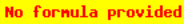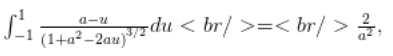This is a test of KaTex, the latex rendering engine used for Khan academy. They advertise themselves as much faster than mathjax, but it looks like the reason for that is because they generate images that look crappy unless the browser resolution is matched to the images just right.

Here’s a rerendering of an old post, with the latex rendered with WP-KaTeX instead of MathJax-LaTeX.

# The post

### Problem:

Calculate the field due to a spherical shell. The field is$\mathbf{E} = \frac{\sigma}{4 \pi \epsilon_0} \int \frac{(\mathbf{r} - \mathbf{r}')}{{{\left\lvert{{\mathbf{r} - \mathbf{r}'}}\right\rvert}}^3} da',$

where$\mathbf{r}'$ is the position to the area element on the shell. For the test position, let$\mathbf{r} = z \mathbf{e}_3$.

### Solution:

We need to parameterize the area integral. A complex-number like geometric algebra representation works nicely.\begin{aligned}\mathbf{r}' &= R \left( \sin\theta \cos\phi, \sin\theta \sin\phi, \cos\theta \right) \\ &= R \left( \mathbf{e}_1 \sin\theta \left( \cos\phi + \mathbf{e}_1 \mathbf{e}_2 \sin\phi \right) + \mathbf{e}_3 \cos\theta \right) \\ &= R \left( \mathbf{e}_1 \sin\theta e^{i\phi} + \mathbf{e}_3 \cos\theta \right).\end{aligned}

Here$i = \mathbf{e}_1 \mathbf{e}_2$ has been used to represent to horizontal rotation plane.

The difference in position between the test vector and area-element is$\mathbf{r} - \mathbf{r}' = \mathbf{e}_3 {\left({ z - R \cos\theta }\right)} - R \mathbf{e}_1 \sin\theta e^{i \phi},$

with an absolute squared length of\begin{aligned}{{\left\lvert{{\mathbf{r} - \mathbf{r}' }}\right\rvert}}^2 &= {\left({ z - R \cos\theta }\right)}^2 + R^2 \sin^2\theta \\ &= z^2 + R^2 - 2 z R \cos\theta.\end{aligned}

As a side note, this is a kind of fun way to prove the old “cosine-law” identity. With that done, the field integral can now be expressed explicitly\begin{aligned} \mathbf{E} &= \frac{\sigma}{4 \pi \epsilon_0} \int_{\phi = 0}^{2\pi} \int_{\theta = 0}^\pi R^2 \sin\theta d\theta d\phi \frac{\mathbf{e}_3 {\left({ z - R \cos\theta }\right)} - R \mathbf{e}_1 \sin\theta e^{i \phi}} { {\left({z^2 + R^2 - 2 z R \cos\theta}\right)}^{3/2} } \\ &= \frac{2 \pi R^2 \sigma \mathbf{e}_3}{4 \pi \epsilon_0} \int_{\theta = 0}^\pi \sin\theta d\theta \frac{z - R \cos\theta} { {\left({z^2 + R^2 - 2 z R \cos\theta}\right)}^{3/2} } \\ &= \frac{2 \pi R^2 \sigma \mathbf{e}_3}{4 \pi \epsilon_0} \int_{\theta = 0}^\pi \sin\theta d\theta \frac{ R( z/R - \cos\theta) } { (R^2)^{3/2} {\left({ (z/R)^2 + 1 - 2 (z/R) \cos\theta}\right)}^{3/2} } \\ &= \frac{\sigma \mathbf{e}_3}{2 \epsilon_0} \int_{u = -1}^{1} du \frac{ z/R - u} { {\left({1 + (z/R)^2 - 2 (z/R) u}\right)}^{3/2} }. \end{aligned}

Observe that all the azimuthal contributions get killed. We expect that due to the symmetry of the problem. We are left with an integral that submits to Mathematica, but doesn’t look fun to attempt manually. Specifically$\int_{-1}^1 \frac{a-u}{{\left({1 + a^2 - 2 a u}\right)}^{3/2}} du = \frac{2}{a^2},$

if$a > 1$, and zero otherwise, so$\boxed{ \mathbf{E} = \frac{\sigma (R/z)^2 \mathbf{e}_3}{\epsilon_0} }$

for$z > R$, and zero otherwise.

In the problem, it is pointed out to be careful of the sign when evaluating$\sqrt{ R^2 + z^2 - 2 R z }$, however, I don’t see where that is even useful?

# KaTex commentary

1. Conditional patterns, such as:

\left\{
\begin{array}{l l}
\frac{\sigma (R/z)^2 \mathbf{e}_3}{\epsilon_0}
& \quad \mbox{if $$z > R$$ } \\
0 & \quad \mbox{if $$z < R$$ }
\end{array}
\right.


messed up KaTex, resulting in render errors like:2. The latex has to be all in one line, or else KaTex renders the newlines explicitly. Example:Having to condense all my latex onto a single line is one of the reasons I switched from the default wordpress latex engine to mathjax. It was annoying enough that I started paying for my wordpress hosting, and stopped posting on my old free peeterjoot.wordpress.com blog. Using KaTex and having to go back to single line latex would suck!
3. The rendering looks like crap, unless you match your resolution to exactly those used to create the images. The mathjax rendering may be slower, but looks much better!
4. The Mathjax-Latex wordpress plugin has some support for equation labeling and references. I don’t see a way to do those with the WP-KaTex plugin.
5. I can have a large set of macros installed in my default.js matching a subset of what I have in my .sty files. I don’t see a way to do that with the WP-KaTex plugin, but perhaps there is just no documented mechanism. KaTex itself does have a macro mechanism.
6. Left justified display mode is hard to read. The mathjax rendered centered display mode looks much better.

## EDIT.

I’m not sure I was getting the katex plugin when I used the [ latex ] … [ /latex ] tags.  I see some comments that indicate that there is built in handling of these tags in the Jetpack plugin.  If I change frontend.php in the katex plugin to use [ katex ] … [ /katex ] tags instead, then I see much different results.